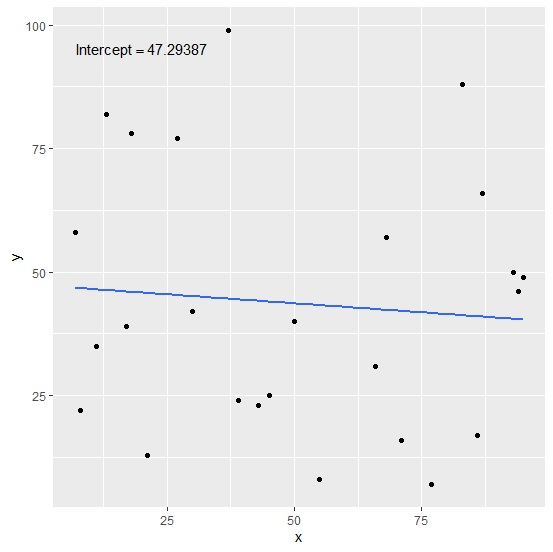# How to display regression intercept using model in a plot created by ggplot2 in R?

To display regression intercept using model in a plot created by ggplot2, we can follow the below steps −

• First of all, create the data frame.
• Use annotate function of ggplot2 to create the scatterplot with regression intercept displayed on the plot.
• Check the regression intercept.

## Create the data frame

Let's create a data frame as shown below −

Live Demo

x<-sample(1:100,25)
y<-sample(1:100,25)
df<-data.frame(x,y)
df

On executing, the above script generates the below output(this output will vary on your system due to randomization) −

  x  y
1 55 8
2 8 22
3 87 66
4 95 49
5 68 57
6 66 31
7 21 13
8 27 77
9 45 25
10 94 46
11 77 7
12 93 50
13 7 58
14 13 82
15 83 88
16 17 39
17 43 23
18 11 35
19 39 24
20 50 40
21 37 99
22 18 78
23 30 42
24 86 17
25 71 16

## Create the scatterplot with regression intercept

Creating the scatterplot with regression line and intercept of the model displayed on the plot −

x<-sample(1:100,25)
y<-sample(1:100,25)
df<-data.frame(x,y)
library(ggplot2)
ggplot(df,aes(x,y))+geom_point()+stat_smooth(method="lm",se=F)+annotate("text",x=2
0,y=95,label=(paste0("Intercept==",coef(lm(df$y~df$x)))),parse=TRUE)
geom_smooth() using formula 'y ~ x'

### Output## Check intercept of the model

Use coeff function to find the intercept of the model and check if it matches with the one displayed in the plot −

Live Demo

x<-sample(1:100,25)
y<-sample(1:100,25)
df<-data.frame(x,y)
coef(lm(df$y~df$x))

### Output

(Intercept)
47.29387